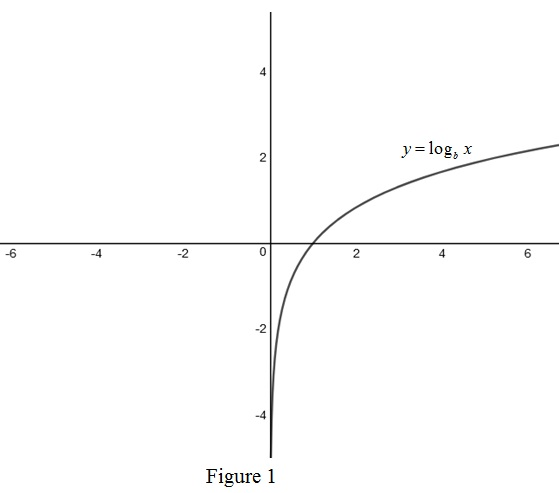# The definition of the logarithmic function y = log b x .### Single Variable Calculus: Concepts...

4th Edition
James Stewart
Publisher: Cengage Learning
ISBN: 9781337687805### Single Variable Calculus: Concepts...

4th Edition
James Stewart
Publisher: Cengage Learning
ISBN: 9781337687805

#### Solutions

Chapter 1.6, Problem 33E

(a)

To determine

## To Explain: The definition of  the logarithmic function y=logbx .

Expert Solution

### Explanation of Solution

Let f(x)=bx be the exponential function and if b>1 , the function is increasing, if 0<b<1 , the function is decreasing.

To prove:

f(x)=f(y) iff  x=y

Proof:

Consider f(x)=bx and f(y)=by

f(x)=f(y)bx=by

Equating the powers on both sides,

x=y

Therefore, it is one to one and b>1 so f1 exists.

Here f1 is called logarithmic function with base b with respect to x.

Thus, the logarithm of x in base b is written for x>0 is given by y=logbx if an only if by=x where x>0 and b>0 .

(b)

To determine

### To find: The domain of y=logbx .

Expert Solution

The domain of y=logbx is the set of positive real numbers.

### Explanation of Solution

Since the logarithmic function is the inverse of the exponential function, the domain of logarithmic function is the range of exponential function.

The exponential function is always positive so logarithmic function is not definedfor negative numbers or for zero.

Therefore, the domain of the logarithmic function y=logbx is the set of positive real numbers.

(c)

To determine

### To find: The range of y=logbx .

Expert Solution

Solution:

The range of y=logbx is set of all real numbers.

### Explanation of Solution

Since the logarithmic function is the inverse of the exponential function, the range of logarithmic function is the domain of exponential function.

The exponential function is defined for all real numbers so logarithmic function can take any real number.

Therefore, the range of the logarithmic function y=logbx is the set of all real numbers.

(d)

To determine

Expert Solution

### Explanation of Solution

Graph:

Use online graph calculator and draw the graph of the function y=logbx if b>1 as shown below in Figure 1.### Have a homework question?

Subscribe to bartleby learn! Ask subject matter experts 30 homework questions each month. Plus, you’ll have access to millions of step-by-step textbook answers!